## Welcome to the Second Life Forums Archive

These forums are CLOSED. Please visit the new forums HERE

# Discussion: Scientific Calculator

Zindorf Yossarian
Master of Disaster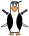Join date: 9 Mar 2004
Posts: 160
06-03-2005 17:03
I just finished a nice calculator script with many nice capabilites, such as trigonometry, parenthesis, and user-defined variables. See the beginning of the script for more details. I say Xylor's Xy Calc, and though it has a bit more function than this, it's also a lot bigger and more complicated!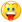So, here goes:
CODE
`// Calculator// By Zindorf Yossarian    (Please include my name with any version of this script - I worked hard on it.)//// CAPABILITES////  My aim with this calculator was to make it capable of most of the basic functions of a scientific calculator. Its capablilies are as follows://    Basics: All the functions addition, subtraction, multiplication, division, exponents, and radicals are supported, as well as negative numbers.//    Parentheses: Any number of parentheses may be used in an expression.//    Trigonometry: Sine, Cosine, Tangent, Arcsine, Arccosine, and Arctangent are supported.//    Angle modes: Answers can computed using degrees or radians.//    pi and e are preset constants, and can be used in your expressions.//    Variables: The user can define and use variables.//    Answer Memory: The calculator will remember the answer to the last problem, and the term "ans" can be used in the next expression to refer to the last answer.//// USING THE CALCULATOR// //  To compute an expression, type the expression, followed by an equals "=" sign. Spacing does not matter.//      ex. "2+2 =" will return 4.  "3^3 =" will return 9.  "3*(4/-2)=" will return -6.//  For radicals, use sqrt. It will take the square root of the value directly following it.//      ex. "sqrt 4 =" will return 2.  "sqrt (20*5) =" will return 10.//  Trigonometric functions too will use the value directly following it.//  The trigonometric functions this calculator uses are sin, cos, tan, asin, acos, & atan.//  asin, acos, and atan are arcfunctions, or 1/sin, 1/cos, and 1/tan. They are the opposite of the sin, cos, and tan.//      ex. "sin 90 =" will return 1. "tan 45 =" will return 1. "atan(tan 30) =" will return 30.//  Angle mode can be toggled between degree and radian mode. To change the mode, say "degree mode" or "radian mode".//  The default mode is degree.//  To store variable, say "store", followed by the desired variable name, followed by the equals "=" sign, followed by the desired variable value.//  The variable name will appear in hovertext, followed by its value. You may also use mathematical expressions to define variables.//      ex. "store foo = 6" will store foo as six, for later use. Text will appear with "foo = 6".//          you may then use foo in an expression: "foo ^ 2 =" will return 36.//          then you may say, "store bar = foo + 3". bar will be stored as 9, and the text will add another line with "bar = 9".//  To delete a variable, say "delete" follwed by the variable name.//  list storedVariables = ["ans", 0, "pi", 3.141593, "e", 2.718282]; // This list is used to store the user-defined variables, and always has three variables for                                   // the last answer "ans" variable, pi, and e. It is strided, so it has the variable name followed by that variable's                                   // value in the list. For now, the value of "ans" is 0, but it will change when expressions are solved.                                   list variableNames = ["ans", "pi", "e"]; // This list holds only the names of the user-defined variables, as well as "ans". It does not include their values.integer angleMode = 1; // angleMode can be either 1 or 0, 1 being degrees mode, and 0 being radian mode. For now we are in degree mode.debug(string debugInput)    // This function exists as a convienient way to debug the script.{                           // All you need to do is pass a string to it, and it will say the string to the owner.    llOwnerSay(debugInput); // This is preferable to many decentralized ownersays, because with this you can stop}                           // the debugs by commenting out one line.update_text() // This function updates the hovertext which displays your variables.{    list unstridedVars; // This list will hold in each entry a string consisting of the variable name, then an equals sign, then the value. We use this list for                        // setting the hovertext that tells the value of each variable.    integer indexCounter; // The indexCounter is used as we scan the strided storedVariables list, and put the names of the variables into the unstridedVars list.        // Remember that list just for holding the variable names? Here's where we put in all those names. First we reset the list to get rid of old, possibly deleted names.    variableNames = ["ans", "pi", "e"];        // We start this loop with the counter at 6, the index in the storedVariables list where the first user-defined variable name is. (remember the first six entries    // are for stored answers and constants) the counter is incremented by 2 each time, so that we go to the next name in the strided list, and skip the numbers.    for (indexCounter = 6; indexCounter < llGetListLength(storedVariables); indexCounter = indexCounter + 2)    {        // We get a list comprising of the variable name and the value after it from the storedVariables list, which is made elsewhere.        // Then we convert those two name and value entries to a string, and insert an "=" between them.        // That string with "name = value" is added to the unstridedVars list.        unstridedVars += [llDumpList2String(llList2List(storedVariables, indexCounter, indexCounter + 1), " = ")];                // Grab the variable name entry from the storedVariables list, and add it to the variableNames list.        variableNames += llList2List(storedVariables, indexCounter, indexCounter);    }        // Finally, the loop finishes, so we can display our variables. The unstridedVars list (with the "name = value" strings) is converted into a string, and "\n" newlines are    // inserted to put each variable on its own line.    llSetText(llDumpList2String(unstridedVars, "\n") + "\n \n \n ", <.7, 0, 0>, 1);        // "What about the variableNames list?", you ask, "You never used it!" Don't worry, we just piggybacked off of this function to set it up. It's used a bit later, in both    // the solving_initiator and the variables_handler functions.}// Here is where it all begins. When the calculator recognizes some speech as a mathematical expression being put in, that expression gets sent here, in raw string form.list solving_initiator(string initiatorInput){    // First things first. We have to convert that big string to something the calculator can understand, like a list. llParseString2List is perfect for this. We can    // look at our string, and make list entries for each mathy thing, while throwing out spaces. But due to some limitations in the number of things the function can parse    // a string into all at once, we divide this parsing business into three parts, converting to strings in between each parsing.        // The first think that gets put into list form is the variables. We parse the string, throwing out nothing, and list-o-fying all the variable names.    list math = llParseString2List(initiatorInput, [], variableNames);    // Now our list probably looks something like this: ["mathy stuff, like 6+2 * sin 3", "some variable name NOT its value.", "some more mathy stuff", "another variable name"]        // The list is put into the variables_handler function, which converts all the variable names to their numerical values. That list is then made into a string, with ", "    // between each element.    string CSVmath = llList2CSV(variables_handler(math));    // And we parse the string again, this time for operators and parenthesis. And we throw out commas, spaces, and that equals sign you put at the end of the expression.    math = llParseString2List(CSVmath, [",", " ","="], ["(", ")", "+", "-", "*", "/", "^"]);    // Now the list looks like this: ["number", "operator", "another number", "another operator", "a variable"]    CSVmath = llList2CSV(math); // Again, the list is converted to a string with ", " between each element.    // Now for the final parsing, for all the trigonometric functions.    math = llParseString2List(CSVmath, [",", " ", "="], ["sqrt", "asin", "acos", "atan", "sin", "cos", "tan"]);        // Yay! we have a list with all the the parts of the expression separated, and with actual numbers instead of variable names.    // It's time to do some math.    // We look for parenthesis in the math list. If there are parentheses, we need to deal with them. If not, we can skip a step and just do the math.    if(llListFindList(math, ["("]) != -1)    {        // Yes, there are parenthesis. Go to the parentheses_handler function, and save whatever it spits back.        math = parentheses_handler(math);    }    else    {        // Nope, no parenthesis. Go to the math_handler function, and save whatever it spits back.        math = math_handler(math);    }        return math; // Finally, return that math list. By now, it should be only one number: The solution.}// This function is for finding the variable names in a list, and replacing them with their value. The function is always called from the solving_initiator,// and is supplied with a list with the variable names parsed out. You will notice that through this function, I just modify the input variable, variablesInput,// until it is what I want to output. Yes, I know this is kinda lame, since most the time it isn't really the input, but why waste extra variables when you already// have a perfectly good one. I do this a lot with input variables, so be aware of it.list variables_handler(list variablesInput){    integer tempInteger; // We use this mostly in the for loop, to look at each part of the input list, checking for variables.    // The loop will consecutively go through each element in the input list.    for (tempInteger = 0; tempInteger < llGetListLength(variablesInput); tempInteger ++)    {        // We look for the current element in the input list, checking to see whether it is in the variables list.        // llListFindList returns -1 if the thing looked for does not exist.        integer tempIndex = llListFindList(variableNames, llList2List(variablesInput, tempInteger, tempInteger));        if (tempIndex != -1)        {            // The index was not -1, so there was a variable! Replace the name with its value.            variablesInput = llListReplaceList(variablesInput, llList2List(storedVariables, tempIndex*2 + 1, tempIndex*2 + 1), tempInteger, tempInteger);        }    }        // The entire input list has been scanned through, so we return the result to the solving_initiator.    return variablesInput;}// The parentheses_handler. It is the backbone of this calculator, and also happens to be the most complicated part. It systematically goes through the expression,// finding sets of parentheses, and handing the contents of them over to the math_handler to be solved. The answer it gets from the math_handler then replaces that// set of parentheses, and it looks for the next set. This is called from the solving_initiator, and takes a fully parsed list with the mathematical expression.list parentheses_handler(list parenInput){    while (TRUE) // We just keep going through this loop over and over until all the parentheses are gone.    {        // We find the index of the first forward parentheses, and the first backward parentheses.        integer forwardParenIndex = llListFindList(parenInput, ["("]);        integer backwardParenIndex = llListFindList(parenInput, [")"]);        if (forwardParenIndex != -1 && forwardParenIndex < backwardParenIndex) // If a forward parenthesis occurs before the backward one.        {            // This finds the backwards parentheses opposite to the forward one.            integer corrParenIndex = corresponding_paren_finder(parenInput, forwardParenIndex, backwardParenIndex);                        // Everything after the forward parentheses is sent to the parentheses_handler, and the result, which will be a single number,  is put into the original            // list, in place of the set of parentheses. Yes, this does call upon itself. Another copy of itself. And that copy might call on another copy, and so on.            // But each time, everything before the first forward parentheses, and the forward parentheses itself is cut off. Eventually, the backwards            // parentheses will come first, or there will be no more forward parentheses. And then that bit of math inside the parentheses is solved, and returned.            // And the next copy solves and returns and the next copy solves and returns until we are all the way back here. If all that hurts your head, don't worry:            // It hurts mine too.            parenInput = llListReplaceList(parenInput, parentheses_handler(llList2List(parenInput, forwardParenIndex + 1, -1)), forwardParenIndex, corrParenIndex);        }        else if (backwardParenIndex != -1 && (forwardParenIndex == -1 || forwardParenIndex > backwardParenIndex)) // There is a backward parentheses, and it is either in        {                                                                                                         // front of the forward one, or there is no forward one.            parenInput = llDeleteSubList(parenInput, backwardParenIndex, -1); // Everything behind and including the backwards parenthesses is deleted.        }        else // If neither of the above two ifs are true, there are no parenthesis. The list is now ripe for the solving.        {            parenInput = math_handler(parenInput); // And solved it is, with the math_handler.            return parenInput; // The solved thing is returned. It should be a single number.        }    }    return ["Something really exploded here."]; // This is just here for the compiler. Obviously, it will never get triggered, there is an "always" loop just above it!}// This function takes a list with a forward parentheses, and finds the backward one that corresponds, then returns the corresponding parentheses' index.// It is also passed the forward and backward parentheses indexes, as found in the parentheses_handler.integer corresponding_paren_finder(list corrParenInput, integer forwardParenIndex, integer backwardParenIndex){    // Since llListFindList only gets the index of the first occurance of something, like our parentheses, we need to replace those parentheses with something else,    // so we can find the next one. Here, we replace forward parentheses with "f" and backwards ones with - you guessed it! - "b". So let's replace the first forward    // parentheses with an "f"...    corrParenInput = llListReplaceList(corrParenInput, ["f"], forwardParenIndex, forwardParenIndex);    forwardParenIndex = llListFindList(corrParenInput, ["("]); // ... and find the next one.    integer level = 0; // "level" is used to keep track of the level or layer of parenthesis we are in, in case parentheses are nested.    do     {        if (forwardParenIndex != -1 && forwardParenIndex < backwardParenIndex) // There is a forward parentheses, and it comes before the backward one. There is a        {                                                                      // nested set of parenthesis!            corrParenInput = llListReplaceList(corrParenInput, ["f"], forwardParenIndex, forwardParenIndex); // Replace that forward parentheses with "f"...            forwardParenIndex = llListFindList(corrParenInput, ["("]); // ... find the next forward parentheses...            level ++; // ... and increase the level        }        else if (level > 0 && (forwardParenIndex == -1 || backwardParenIndex < forwardParenIndex)) // There is a backwards parenthesis before any forward ones, and         {                                                                                          // the level is greater than 0. We need to check the level so that if                                                                                                   // this is the last backwards parentheses we don't replace it, thereby                                                                                                   // losing the index.            corrParenInput = llListReplaceList(corrParenInput, ["b"], backwardParenIndex, backwardParenIndex); // Replace the parentheses with a "b"...            backwardParenIndex = llListFindList(corrParenInput, [")"]); // ... find the next one...            level --; // ... and decrease the level.        }    } while (level > 0); // Now, after all that is done, the level is checked. If is is more than zero, it repeats, or else it breaks from the loop.    return(llListFindList(corrParenInput, [")"])); // Return the index of the corresponding backwards parentheses.}// Ahh, the math_handler! So good for doing... math. This will take a list, which is by now free of parenthesis and variables, and is just numbers and operators,// and computes the expression's value, using the proper order of operations.list math_handler(list mathInput){    integer tempIndex; // tempIndex is used a lot, in the many "for" loops here, as the index of an operator.    float tempSolution; // the solution to each small bit of the expression is put here for about .001 seconds, and then is moved into the main mathInput list, which                        // slowly but surely gets solved.                            // Each part of the math_handler represents a different calculator function. They all use "for" loops to check for the existence and location of the operator    // or trig function. Many are almost exactly similar to each other. The stuff inside the loop will only happen if the operator it is looking for exists.        // negatives - this looks for each "-", and then checks out what comes before it to see whether it should be a negative number or a minus sign.    for (tempIndex = llListFindList(mathInput, ["-"]); tempIndex != -1; tempIndex = llListFindList(mathInput, ["-"]))    {        // If the "-" appears before anything else, or an operator appears before it, it must be a negative.        if (tempIndex == 0 || -1 != llListFindList(["(", "*", "/", "^", "sqrt", "sin", "cos", "tan", "asin", "acos", "atan", "+", "-"], llList2List(mathInput, tempIndex - 1, tempIndex - 1)))        {            tempSolution = (-1 * (float)llList2String(mathInput, tempIndex + 1)); // So multiply the number after the "-" by negative one...            mathInput = llListReplaceList(mathInput, [tempSolution], tempIndex, tempIndex + 1); // ... and replace both the "-" and the number with that negative.        }        else // It's a minus sign, but we still replace it with "m", to distinguish it from negatives. Remember, that "for" loop checks for "-" each time. If we don't        {    // replace the "-" with something else, it will loop endlessly.            mathInput = llListReplaceList(mathInput, ["m"], tempIndex, tempIndex);        }    }    // sines    for (tempIndex = llListFindList(mathInput, ["sin"]); tempIndex != -1; tempIndex = llListFindList(mathInput, ["sin"]))    {        // This is a compressed if/else statement, to save space. It uses an if/else to provide an answer in radians or degrees, depending on the mode.        // If the value of mode is 1, then input is converted to degrees before being processed. Or, if mode equals 0, no conversion occurs.        // The sin of the list element after the "sin" in the list is found, and that answer is put into the mathInput list. The next 5 trig functions        // are all roughly the same, so I won't bother explaining them.        if (angleMode) { tempSolution = llSin((float)llList2String(mathInput, tempIndex + 1) * DEG_TO_RAD); }         else { tempSolution = llSin((float)llList2String(mathInput, tempIndex + 1)); }        mathInput = llListReplaceList(mathInput, [tempSolution], tempIndex, tempIndex + 1);    }    // cosines    for (tempIndex = llListFindList(mathInput, ["cos"]); tempIndex != -1; tempIndex = llListFindList(mathInput, ["cos"]))    {        if (angleMode) { tempSolution = llCos((float)llList2String(mathInput, tempIndex + 1) * DEG_TO_RAD); }        else { tempSolution = llCos((float)llList2String(mathInput, tempIndex + 1)); }        mathInput = llListReplaceList(mathInput, [tempSolution], tempIndex, tempIndex + 1);    }    // tangents    for (tempIndex = llListFindList(mathInput, ["tan"]); tempIndex != -1; tempIndex = llListFindList(mathInput, ["tan"]))    {        if (angleMode) { tempSolution = llTan((float)llList2String(mathInput, tempIndex + 1) * DEG_TO_RAD); }        else { tempSolution = llTan((float)llList2String(mathInput, tempIndex + 1)); }        mathInput = llListReplaceList(mathInput, [tempSolution], tempIndex, tempIndex + 1);    }    // arcsines    for (tempIndex = llListFindList(mathInput, ["asin"]); tempIndex != -1; tempIndex = llListFindList(mathInput, ["asin"]))    {        if (angleMode) { tempSolution = llAsin((float)llList2String(mathInput, tempIndex + 1))  * RAD_TO_DEG; }        else { tempSolution = llAsin((float)llList2String(mathInput, tempIndex + 1)); }        mathInput = llListReplaceList(mathInput, [tempSolution], tempIndex, tempIndex + 1);    }    // arccosines    for (tempIndex = llListFindList(mathInput, ["acos"]); tempIndex != -1; tempIndex = llListFindList(mathInput, ["acos"]))    {        if (angleMode) { tempSolution = llAcos((float)llList2String(mathInput, tempIndex + 1))  * RAD_TO_DEG; }        else { tempSolution = llAcos((float)llList2String(mathInput, tempIndex + 1)); }        mathInput = llListReplaceList(mathInput, [tempSolution], tempIndex, tempIndex + 1);    }    // arctangents    for (tempIndex = llListFindList(mathInput, ["atan"]); tempIndex != -1; tempIndex = llListFindList(mathInput, ["atan"]))    {        if (angleMode) { tempSolution = llAtan2((float)llList2String(mathInput, tempIndex + 1), 1)  * RAD_TO_DEG; }        else { tempSolution = llAtan2((float)llList2String(mathInput, tempIndex + 1), 1); }        mathInput = llListReplaceList(mathInput, [tempSolution], tempIndex, tempIndex + 1);    }    // squareroots    for (tempIndex = llListFindList(mathInput, ["sqrt"]); tempIndex != -1; tempIndex = llListFindList(mathInput, ["sqrt"]))    {        tempSolution = llSqrt((float)llList2String(mathInput, tempIndex + 1));              // This finds the squareroot of the number after "sqrt",        mathInput = llListReplaceList(mathInput, [tempSolution], tempIndex, tempIndex + 1); // and puts it into the mathInput list.    }    // exponents    for (tempIndex = llListFindList(mathInput, ["^"]); tempIndex != -1; tempIndex = llListFindList(mathInput, ["^"]))    {        tempSolution = llPow((float)llList2String(mathInput, tempIndex - 1) , (float)llList2String(mathInput, tempIndex + 1)); // This finds the number before "^"        mathInput = llListReplaceList(mathInput, [tempSolution], tempIndex - 1, tempIndex + 1);                                // to the power of the number after,    }                                                                                                                          // and puts it into the mathInput list.    // multiplication    for (tempIndex = llListFindList(mathInput, ["*"]); tempIndex != -1; tempIndex = llListFindList(mathInput, ["*"]))    {        tempSolution = (float)llList2String(mathInput, tempIndex - 1) * (float)llList2String(mathInput, tempIndex + 1); // Multiplies the number before "*"        mathInput = llListReplaceList(mathInput, [tempSolution], tempIndex - 1, tempIndex + 1);                         // by the number after, and puts it into    }                                                                                                                   // the mathInput list.    // division    for (tempIndex = llListFindList(mathInput, ["/"]); tempIndex != -1; tempIndex = llListFindList(mathInput, ["/"]))    {        tempSolution = (float)llList2String(mathInput, tempIndex - 1) / (float)llList2String(mathInput, tempIndex + 1); // Follows the same format as multiplication        mathInput = llListReplaceList(mathInput, [tempSolution], tempIndex - 1, tempIndex + 1);    }    // addition    for (tempIndex = llListFindList(mathInput, ["+"]); tempIndex != -1; tempIndex = llListFindList(mathInput, ["+"]))    {        tempSolution = (float)llList2String(mathInput, tempIndex - 1) + (float)llList2String(mathInput, tempIndex + 1);  // Follows the same format as division        mathInput = llListReplaceList(mathInput, [tempSolution], tempIndex - 1, tempIndex + 1);    }    // subtraction    for (tempIndex = llListFindList(mathInput, ["m"]); tempIndex != -1; tempIndex = llListFindList(mathInput, ["m"]))    {        tempSolution = (float)llList2String(mathInput, tempIndex - 1) - (float)llList2String(mathInput, tempIndex + 1);  // Follows the same format as addition        mathInput = llListReplaceList(mathInput, [tempSolution], tempIndex - 1, tempIndex + 1);    }    // We have now gone through the list and solved everything. There is one number left in the list.    return mathInput;}// That's all the functions. Now into the friendly old default state.default{    state_entry()    {        llSetText("", <0,0,0>, 0); // Clears all hovertext with the variables.        llListen(0, "", llGetOwner(), ""); // Listens to the owner.    }    listen(integer channel, string name, key id, string message)    {        if (llGetSubString(message, -1, -1) == "=") // If an "=" sign comes last in the message, the user wants us to compute something!        {            list math = solving_initiator(message); // Send the message all the way up to the solving_initiator, where it begins its long journey to solvedom.                                                    // After said long journey, the answer is put into the math list.                        llWhisper(0, llDumpList2String(math, " ")); // Whisper the answer. (You could use ownersay, but then you can't show off the calculator.)                        storedVariables = llListReplaceList(storedVariables, [llList2Float(math, 0)], 1, 1); // We just got a new answer, so put that in for the value of "ans"                    }        else if (llGetSubString(message, 0, 4) == "store") // The user wants to store something.        {            integer equalsIndex = llSubStringIndex(message, "="); // Get the index of the equals sign.                        // Get whatever comes after the equals sign, and solve it. Then store that solution to the variableValue list.            list variableValue = solving_initiator(llGetSubString(message, equalsIndex + 2, -1));            integer tempIndex = llListFindList(storedVariables, [llGetSubString(message, 6, equalsIndex - 2)]); // See if the variable is already stored.            if (tempIndex == -1) // If it is not...            {                storedVariables = storedVariables + [llGetSubString(message, 6, equalsIndex - 2), llList2String(variableValue, 0)]; // Add the variable and its value to the list.                update_text(); // Update the hovertext. (and the variableNames list too.)            }            else if (tempIndex > 7) // The variable was already in the list, and is not "ans" or a constant...            {                   // So we just replace the old value with the new one                storedVariables = llListReplaceList(storedVariables, [llList2String(variableValue, 0)], tempIndex + 1, tempIndex + 1);            }        }        else if (llGetSubString(message, 0, 5) == "delete") // The user wants to delete a variable.        {            integer tempIndex = llListFindList(storedVariables, [llGetSubString(message, 7, -1)]); // Find where that variable is            if (tempIndex > 5) // If the variable is in the list, and is not the answer variable or a constant...            {                storedVariables = llDeleteSubList(storedVariables, tempIndex, tempIndex + 1); // Delete the name and value of the variable                update_text(); // And update the text and variableNames            }        }        else if (llGetSubString(message, -4, -1) == "mode") // The user wants to change the mode.        {            if (llGetSubString(message, 0, -6) == "radian") // And he wants radian mode.            {                llWhisper(0, "Calculator set to radian mode."); // Acknowledge the change, so the user knows the calculator heard him.                angleMode = 0; // And change the mode integer to 0. Since radians are used by the LSL functions, no conversion will occur            }            else if (llGetSubString(message, 0, -6) == "degree") // He wants degree mode.            {                llWhisper(0, "Calculator set to degree mode."); // Acknowledge the change.                angleMode = 1; // Change the mode integer so that the angles will be converted.            }        }    }}// Whew! All done commenting.`
_____________________
Badass Ninja Penguin: Killing stuff it doesn't like since sometime in May 2004.
Nada Epoch
The Librarian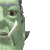Join date: 4 Nov 2002
Posts: 1,423
Original thread
06-04-2005 08:21
/15/88/49018/1.html
_____________________
i've got nothing.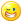Keknehv Psaltery
Hacker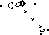Join date: 11 Apr 2005
Posts: 1,185
07-23-2005 11:19
This thing is actually messed up... It doesn't follow the order of operations.

Here's an example. Tell the calculator to find the value of this expression: "6/3*2". Now, if it followed the order of operations properly, it would do 6/3 first, yielding 2, and then would multiply that by 2, yielding 4. However, this calculator thinks that the expression is equal to 1.

It doesn't follow the order with exponents and radicals, either, nor with the trig functions.
_____________________
Vortex Saito
Quintzee Creator
Join date: 10 Sep 2004
Posts: 73
07-23-2005 15:49
Hmmm in my rule book multipling comes before dividing.
_____________________
I don't care I am a lemming, I am NOT going !!!!

secondlife://puea/54/15
Pete Fats
Geek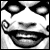Join date: 18 Apr 2003
Posts: 648
07-23-2005 15:57
Please excuse my dear aunt Sally.
_____________________Eloise Pasteur
Curious Individual
Join date: 14 Jul 2004
Posts: 1,952
07-23-2005 18:01
From: Vortex Saito
Hmmm in my rule book multipling comes before dividing.

Speaking as a maths teacher can I suggest the order should be:

Brackets
Powers of
Mulitplying/Dividing (equal so use Left to Right)
Addition/Subtraction (equal so use Left to Right)

So 6/3*2=4 because the multiplying and dividing are equally important so you apply the left to right when solving.
Keknehv Psaltery
HackerJoin date: 11 Apr 2005
Posts: 1,185
07-23-2005 21:59
Actually, it works if you do division first and then multiplication.

PEDMAS

Parentheses, Exponents, Division, Multiplication, Addition, Subtraction

Just try an find a problem where you would get a different answer with this order than with the left-to-right.
_____________________
Champie Jack
Registered User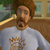Join date: 6 Dec 2003
Posts: 1,156
07-24-2005 02:57
if you look at the script, after all parentheses are calculated and all exponents, the math handler just goes in order with if statements..and multiplication comes before division. the same happens with addition and subtraction...it always computes addition before subtraction...it's built into the logic.

here's the snippet from the math_handler:
CODE
`    // multiplication    for (tempIndex = llListFindList(mathInput, ["*"]); tempIndex != -1; tempIndex = llListFindList(mathInput, ["*"]))    {        tempSolution = (float)llList2String(mathInput, tempIndex - 1) * (float)llList2String(mathInput, tempIndex + 1); // Multiplies the number before "*"        mathInput = llListReplaceList(mathInput, [tempSolution], tempIndex - 1, tempIndex + 1);                         // by the number after, and puts it into    }                                                                                                                   // the mathInput list.    // division    for (tempIndex = llListFindList(mathInput, ["/"]); tempIndex != -1; tempIndex = llListFindList(mathInput, ["/"]))    {        tempSolution = (float)llList2String(mathInput, tempIndex - 1) / (float)llList2String(mathInput, tempIndex + 1); // Follows the same format as multiplication        mathInput = llListReplaceList(mathInput, [tempSolution], tempIndex - 1, tempIndex + 1);    }    // addition    for (tempIndex = llListFindList(mathInput, ["+"]); tempIndex != -1; tempIndex = llListFindList(mathInput, ["+"]))    {        tempSolution = (float)llList2String(mathInput, tempIndex - 1) + (float)llList2String(mathInput, tempIndex + 1);  // Follows the same format as division        mathInput = llListReplaceList(mathInput, [tempSolution], tempIndex - 1, tempIndex + 1);    }    // subtraction    for (tempIndex = llListFindList(mathInput, ["m"]); tempIndex != -1; tempIndex = llListFindList(mathInput, ["m"]))    {        tempSolution = (float)llList2String(mathInput, tempIndex - 1) - (float)llList2String(mathInput, tempIndex + 1);  // Follows the same format as addition        mathInput = llListReplaceList(mathInput, [tempSolution], tempIndex - 1, tempIndex + 1);    }  `

It simply computes multiplication before division and addition before subtraction.

If you can easily fix that, then you are good to go.

I haven't spent any time to figure out how all the lists work and what they look like once they get to the math_handler. I' plan on spending a few minutes with it, but I'm sure there is someone infinitely more capable than me who could sort it out in no time.

Champie
_____________________
Champie Jack
Registered UserJoin date: 6 Dec 2003
Posts: 1,156
07-24-2005 02:58
From: Keknehv Psaltery
Actually, it works if you do division first and then multiplication.

PEDMAS

Parentheses, Exponents, Division, Multiplication, Addition, Subtraction

Just try an find a problem where you would get a different answer with this order than with the left-to-right.

So are you saying we just need to switch the if statements and it will work fine?
_____________________
Vortex Saito
Quintzee Creator
Join date: 10 Sep 2004
Posts: 73
07-24-2005 03:01
From: Eloise Pasteur
Speaking as a maths teacher can I suggest the order should be:

Brackets
Powers of
Mulitplying/Dividing (equal so use Left to Right)
Addition/Subtraction (equal so use Left to Right)

So 6/3*2=4 because the multiplying and dividing are equally important so you apply the left to right when solving.

<Bows head in shame>

Yes Teach, I know, I shouldn't post in forums when it's actually time for me to go to bed
_____________________
I don't care I am a lemming, I am NOT going !!!!

secondlife://puea/54/15
Champie Jack
Registered UserJoin date: 6 Dec 2003
Posts: 1,156
07-24-2005 03:55
ok, I think I fixed it

this is the fix:
CODE
`        //**************************************************************** ORDER OF OPERATIONS FIX **********************************************************//                    listLength = llGetListLength(mathInput);            string tempstring;            for (x = 0; x < (listLength); x++)            {                tempstring =  llList2String(mathInput, x);                if (tempstring == "*")                {                    tempSolution = (float)llList2String(mathInput, x - 1) * (float)llList2String(mathInput, x + 1);                    mathInput = llListReplaceList(mathInput, [tempSolution], x - 1, x + 1);                    x = 0;                }                if (tempstring == "/")                {                    tempSolution = (float)llList2String(mathInput, x - 1) / (float)llList2String(mathInput, x + 1);                    mathInput = llListReplaceList(mathInput, [tempSolution], x - 1, x + 1);                    x = 0;                }                if (tempstring == "+")                {                    llWhisper(0, "x= " +(string)x + " value = " + llList2String(mathInput, x));                    tempSolution = (float)llList2String(mathInput, x - 1) + (float)llList2String(mathInput, x + 1);                    mathInput = llListReplaceList(mathInput, [tempSolution], x - 1, x + 1);                    x = 0;                }                if (tempstring == "m")                {                    tempSolution = (float)llList2String(mathInput, x - 1) - (float)llList2String(mathInput, x + 1);                    mathInput = llListReplaceList(mathInput, [tempSolution], x - 1, x + 1);                    x = 0;                }                listLength = llGetListLength(mathInput);            }                                                                                                                                                                                                   // We have now gone through the list and solved everything. There is one number left in the list.        return mathInput;                 //**************************************************************** ORDER OF OPERATIONS FIX **********************************************************//`

And it replaces everything from "//multiplication" to "return mathInput"

here is the snippet that gets removed:
CODE
`    // multiplication    for (tempIndex = llListFindList(mathInput, ["*"]); tempIndex != -1; tempIndex = llListFindList(mathInput, ["*"]))    {        tempSolution = (float)llList2String(mathInput, tempIndex - 1) * (float)llList2String(mathInput, tempIndex + 1); // Multiplies the number before "*"        mathInput = llListReplaceList(mathInput, [tempSolution], tempIndex - 1, tempIndex + 1);                         // by the number after, and puts it into    }                                                                                                                   // the mathInput list.    // division    for (tempIndex = llListFindList(mathInput, ["/"]); tempIndex != -1; tempIndex = llListFindList(mathInput, ["/"]))    {        tempSolution = (float)llList2String(mathInput, tempIndex - 1) / (float)llList2String(mathInput, tempIndex + 1); // Follows the same format as multiplication        mathInput = llListReplaceList(mathInput, [tempSolution], tempIndex - 1, tempIndex + 1);    }    // addition    for (tempIndex = llListFindList(mathInput, ["+"]); tempIndex != -1; tempIndex = llListFindList(mathInput, ["+"]))    {        tempSolution = (float)llList2String(mathInput, tempIndex - 1) + (float)llList2String(mathInput, tempIndex + 1);  // Follows the same format as division        mathInput = llListReplaceList(mathInput, [tempSolution], tempIndex - 1, tempIndex + 1);    }    // subtraction    for (tempIndex = llListFindList(mathInput, ["m"]); tempIndex != -1; tempIndex = llListFindList(mathInput, ["m"]))    {        tempSolution = (float)llList2String(mathInput, tempIndex - 1) - (float)llList2String(mathInput, tempIndex + 1);  // Follows the same format as addition        mathInput = llListReplaceList(mathInput, [tempSolution], tempIndex - 1, tempIndex + 1);    }    // We have now gone through the list and solved everything. There is one number left in the list.    return mathInput;`
_____________________
Champie Jack
Registered UserJoin date: 6 Dec 2003
Posts: 1,156
07-24-2005 04:05
ok, here's the fix

I actually had already posted it, but I had made an error, so I deleted the post..but here it is for real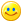CODE
`list storedVariables = ["ans", 0, "pi", 3.141593, "e", 2.718282]; // This list is used to store the user-defined variables, and always has three variables for                                   // the last answer "ans" variable, pi, and e. It is strided, so it has the variable name followed by that variable's                                   // value in the list. For now, the value of "ans" is 0, but it will change when expressions are solved.                                   list variableNames = ["ans", "pi", "e"]; // This list holds only the names of the user-defined variables, as well as "ans". It does not include their values.integer angleMode = 1; // angleMode can be either 1 or 0, 1 being degrees mode, and 0 being radian mode. For now we are in degree mode.debug(string debugInput)    // This function exists as a convienient way to debug the script.{                           // All you need to do is pass a string to it, and it will say the string to the owner.    llOwnerSay(debugInput); // This is preferable to many decentralized ownersays, because with this you can stop}                           // the debugs by commenting out one line.update_text() // This function updates the hovertext which displays your variables.{    list unstridedVars; // This list will hold in each entry a string consisting of the variable name, then an equals sign, then the value. We use this list for                        // setting the hovertext that tells the value of each variable.    integer indexCounter; // The indexCounter is used as we scan the strided storedVariables list, and put the names of the variables into the unstridedVars list.        // We start this loop with the counter at 6, the index in the storedVariables list where the first user-defined variable name is. (remember the first six entries    // are for stored answers and constants) the counter is incremented by 2 each time, so that we go to the next name in the strided list, and skip the numbers.    for (indexCounter = 6; indexCounter < llGetListLength(storedVariables); indexCounter = indexCounter + 2)    {        // We get a list comprising of the variable name and the value after it from the storedVariables list, which is made elsewhere.        // Then we convert those two name and value entries to a string, and insert an "=" between them.        // That string with "name = value" is added to the unstridedVars list.        unstridedVars += [llDumpList2String(llList2List(storedVariables, indexCounter, indexCounter + 1), " = ")];                // Remember that list just for holding the variable names? Here's where we put in all those names. First we reset the list to get rid of old, possibly deleted names.        variableNames = ["ans", "pi", "e"];        // Now that we have a nice clean slate, we just grab the variable name entry from the storedVariables list, and add it to the variableNames list.        variableNames += llList2List(storedVariables, indexCounter, indexCounter);    }        // Finally, the loop finishes, so we can display our variables. The unstridedVars list (with the "name = value" strings) is converted into a string, and "\n" newlines are    // inserted to put each variable on its own line.    llSetText(llDumpList2String(unstridedVars, "\n") + "\n \n \n ", <.7, 0, 0>, 1);        // "What about the variableNames list?", you ask, "You never used it!" Don't worry, we just piggybacked off of this function to set it up. It's used a bit later, in both    // the solving_initiator and the variables_handler functions.}// Here is where it all begins. When the calculator recognizes some speech as a mathematical expression being put in, that expression gets sent here, in raw string form.list solving_initiator(string initiatorInput){    // First things first. We have to convert that big string to something the calculator can understand, like a list. llParseString2List is perfect for this. We can    // look at our string, and make list entries for each mathy thing, while throwing out spaces. But due to some limitations in the number of things the function can parse    // a string into all at once, we divide this parsing business into three parts, converting to strings in between each parsing.        // The first think that gets put into list form is the variables. We parse the string, throwing out nothing, and list-o-fying all the variable names.    list math = llParseString2List(initiatorInput, [], variableNames);    // Now our list probably looks something like this: ["mathy stuff, like 6+2 * sin 3", "some variable name NOT its value.", "some more mathy stuff", "another variable name"]        // The list is put into the variables_handler function, which converts all the variable names to their numerical values. That list is then made into a string, with ", "    // between each element.    string CSVmath = llList2CSV(variables_handler(math));    // And we parse the string again, this time for operators and parenthesis. And we throw out commas, spaces, and that equals sign you put at the end of the expression.    math = llParseString2List(CSVmath, [",", " ","="], ["(", ")", "+", "-", "*", "/", "^"]);    // Now the list looks like this: ["number", "operator", "another number", "another operator", "a variable"]    CSVmath = llList2CSV(math); // Again, the list is converted to a string with ", " between each element.    // Now for the final parsing, for all the trigonometric functions.    math = llParseString2List(CSVmath, [",", " ", "="], ["sqrt", "asin", "acos", "atan", "sin", "cos", "tan"]);        // Yay! we have a list with all the the parts of the expression separated, and with actual numbers instead of variable names.    // It's time to do some math.    // We look for parenthesis in the math list. If there are parentheses, we need to deal with them. If not, we can skip a step and just do the math.    if(llListFindList(math, ["("]) != -1)    {        // Yes, there are parenthesis. Go to the parentheses_handler function, and save whatever it spits back.        math = parentheses_handler(math);    }    else    {        // Nope, no parenthesis. Go to the math_handler function, and save whatever it spits back.        math = math_handler(math);    }        return math; // Finally, return that math list. By now, it should be only one number: The solution.}// This function is for finding the variable names in a list, and replacing them with their value. The function is always called from the solving_initiator,// and is supplied with a list with the variable names parsed out. You will notice that through this function, I just modify the input variable, variablesInput,// until it is what I want to output. Yes, I know this is kinda lame, since most the time it isn't really the input, but why waste extra variables when you already// have a perfectly good one. I do this a lot with input variables, so be aware of it.list variables_handler(list variablesInput){    integer tempInteger; // We use this mostly in the for loop, to look at each part of the input list, checking for variables.    // The loop will consecutively go through each element in the input list.    for (tempInteger = 0; tempInteger < llGetListLength(variablesInput); tempInteger ++)    {        // We look for the current element in the input list, checking to see whether it is in the variables list.        // llListFindList returns -1 if the thing looked for does not exist.        integer tempIndex = llListFindList(variableNames, llList2List(variablesInput, tempInteger, tempInteger));        if (tempIndex != -1)        {            // The index was not -1, so there was a variable! Replace the name with its value.            variablesInput = llListReplaceList(variablesInput, llList2List(storedVariables, tempIndex*2 + 1, tempIndex*2 + 1), tempInteger, tempInteger);        }    }        // The entire input list has been scanned through, so we return the result to the solving_initiator.    return variablesInput;}// The parentheses_handler. It is the backbone of this calculator, and also happens to be the most complicated part. It systematically goes through the expression,// finding sets of parentheses, and handing the contents of them over to the math_handler to be solved. The answer it gets from the math_handler then replaces that// set of parentheses, and it looks for the next set. This is called from the solving_initiator, and takes a fully parsed list with the mathematical expression.list parentheses_handler(list parenInput){    while (TRUE) // We just keep going through this loop over and over until all the parentheses are gone.    {        // We find the index of the first forward parentheses, and the first backward parentheses.        integer forwardParenIndex = llListFindList(parenInput, ["("]);        integer backwardParenIndex = llListFindList(parenInput, [")"]);        if (forwardParenIndex != -1 && forwardParenIndex < backwardParenIndex) // If a forward parenthesis occurs before the backward one.        {            // This finds the backwards parentheses opposite to the forward one.            integer corrParenIndex = corresponding_paren_finder(parenInput, forwardParenIndex, backwardParenIndex);                        // Everything after the forward parentheses is sent to the parentheses_handler, and the result, which will be a single number,  is put into the original            // list, in place of the set of parentheses. Yes, this does call upon itself. Another copy of itself. And that copy might call on another copy, and so on.            // But each time, everything before the first forward parentheses, and the forward parentheses itself is cut off. Eventually, the backwards            // parentheses will come first, or there will be no more forward parentheses. And then that bit of math inside the parentheses is solved, and returned.            // And the next copy solves and returns and the next copy solves and returns until we are all the way back here. If all that hurts your head, don't worry:            // It hurts mine too.            parenInput = llListReplaceList(parenInput, parentheses_handler(llList2List(parenInput, forwardParenIndex + 1, -1)), forwardParenIndex, corrParenIndex);        }        else if (backwardParenIndex != -1 && (forwardParenIndex == -1 || forwardParenIndex > backwardParenIndex)) // There is a backward parentheses, and it is either in        {                                                                                                         // front of the forward one, or there is no forward one.            parenInput = llDeleteSubList(parenInput, backwardParenIndex, -1); // Everything behind and including the backwards parenthesses is deleted.        }        else // If neither of the above two ifs are true, there are no parenthesis. The list is now ripe for the solving.        {            parenInput = math_handler(parenInput); // And solved it is, with the math_handler.            return parenInput; // The solved thing is returned. It should be a single number.        }    }    return ["Something really exploded here."]; // This is just here for the compiler. Obviously, it will never get triggered, there is an "always" loop just above it!}// This function takes a list with a forward parentheses, and finds the backward one that corresponds, then returns the corresponding parentheses' index.// It is also passed the forward and backward parentheses indexes, as found in the parentheses_handler.integer corresponding_paren_finder(list corrParenInput, integer forwardParenIndex, integer backwardParenIndex){    // Since llListFindList only gets the index of the first occurance of something, like our parentheses, we need to replace those parentheses with something else,    // so we can find the next one. Here, we replace forward parentheses with "f" and backwards ones with - you guessed it! - "b". So let's replace the first forward    // parentheses with an "f"...    corrParenInput = llListReplaceList(corrParenInput, ["f"], forwardParenIndex, forwardParenIndex);    forwardParenIndex = llListFindList(corrParenInput, ["("]); // ... and find the next one.    integer level = 0; // "level" is used to keep track of the level or layer of parenthesis we are in, in case parentheses are nested.    do     {        if (forwardParenIndex != -1 && forwardParenIndex < backwardParenIndex) // There is a forward parentheses, and it comes before the backward one. There is a        {                                                                      // nested set of parenthesis!            corrParenInput = llListReplaceList(corrParenInput, ["f"], forwardParenIndex, forwardParenIndex); // Replace that forward parentheses with "f"...            forwardParenIndex = llListFindList(corrParenInput, ["("]); // ... find the next forward parentheses...            level ++; // ... and increase the level        }        else if (level > 0 && (forwardParenIndex == -1 || backwardParenIndex < forwardParenIndex)) // There is a backwards parenthesis before any forward ones, and         {                                                                                          // the level is greater than 0. We need to check the level so that if                                                                                                   // this is the last backwards parentheses we don't replace it, thereby                                                                                                   // losing the index.            corrParenInput = llListReplaceList(corrParenInput, ["b"], backwardParenIndex, backwardParenIndex); // Replace the parentheses with a "b"...            backwardParenIndex = llListFindList(corrParenInput, [")"]); // ... find the next one...            level --; // ... and decrease the level.        }    } while (level > 0); // Now, after all that is done, the level is checked. If is is more than zero, it repeats, or else it breaks from the loop.    return(llListFindList(corrParenInput, [")"])); // Return the index of the corresponding backwards parentheses.}// Ahh, the math_handler! So good for doing... math. This will take a list, which is by now free of parenthesis and variables, and is just numbers and operators,// and computes the expression's value, using the proper order of operations.list math_handler(list mathInput){    integer listLength = llGetListLength(mathInput);    //llWhisper(0, (string)listLength);    integer x;    for (x = 0; x < (listLength); x++)    {        //llWhisper(0, llList2String(mathInput, x));    }        integer tempIndex; // tempIndex is used a lot, in the many "for" loops here, as the index of an operator.        float tempSolution; // the solution to each small bit of the expression is put here for about .001 seconds, and then is moved into the main mathInput list, which                            // slowly but surely gets solved.                                    // Each part of the math_handler represents a different calculator function. They all use "for" loops to check for the existence and location of the operator        // or trig function. Many are almost exactly similar to each other. The stuff inside the loop will only happen if the operator it is looking for exists.                // negatives - this looks for each "-", and then checks out what comes before it to see whether it should be a negative number or a minus sign.        for (tempIndex = llListFindList(mathInput, ["-"]); tempIndex != -1; tempIndex = llListFindList(mathInput, ["-"]))        {            // If the "-" appears before anything else, or an operator appears before it, it must be a negative.            if (tempIndex == 0 || -1 != llListFindList(["(", "*", "/", "^", "sqrt", "sin", "cos", "tan", "asin", "acos", "atan", "+", "-"], llList2List(mathInput, tempIndex - 1, tempIndex - 1)))            {                tempSolution = (-1 * (float)llList2String(mathInput, tempIndex + 1)); // So multiply the number after the "-" by negative one...                mathInput = llListReplaceList(mathInput, [tempSolution], tempIndex, tempIndex + 1); // ... and replace both the "-" and the number with that negative.            }            else // It's a minus sign, but we still replace it with "m", to distinguish it from negatives. Remember, that "for" loop checks for "-" each time. If we don't            {    // replace the "-" with something else, it will loop endlessly.                mathInput = llListReplaceList(mathInput, ["m"], tempIndex, tempIndex);            }        }        // sines        for (tempIndex = llListFindList(mathInput, ["sin"]); tempIndex != -1; tempIndex = llListFindList(mathInput, ["sin"]))        {            // This is a compressed if/else statement, to save space. It uses an if/else to provide an answer in radians or degrees, depending on the mode.            // If the value of mode is 1, then input is converted to degrees before being processed. Or, if mode equals 0, no conversion occurs.            // The sin of the list element after the "sin" in the list is found, and that answer is put into the mathInput list. The next 5 trig functions            // are all roughly the same, so I won't bother explaining them.            if (angleMode) { tempSolution = llSin((float)llList2String(mathInput, tempIndex + 1) * DEG_TO_RAD); }             else { tempSolution = llSin((float)llList2String(mathInput, tempIndex + 1)); }            mathInput = llListReplaceList(mathInput, [tempSolution], tempIndex, tempIndex + 1);        }        // cosines        for (tempIndex = llListFindList(mathInput, ["cos"]); tempIndex != -1; tempIndex = llListFindList(mathInput, ["cos"]))        {            if (angleMode) { tempSolution = llCos((float)llList2String(mathInput, tempIndex + 1) * DEG_TO_RAD); }            else { tempSolution = llCos((float)llList2String(mathInput, tempIndex + 1)); }            mathInput = llListReplaceList(mathInput, [tempSolution], tempIndex, tempIndex + 1);        }        // tangents        for (tempIndex = llListFindList(mathInput, ["tan"]); tempIndex != -1; tempIndex = llListFindList(mathInput, ["tan"]))        {            if (angleMode) { tempSolution = llTan((float)llList2String(mathInput, tempIndex + 1) * DEG_TO_RAD); }            else { tempSolution = llTan((float)llList2String(mathInput, tempIndex + 1)); }            mathInput = llListReplaceList(mathInput, [tempSolution], tempIndex, tempIndex + 1);        }        // arcsines        for (tempIndex = llListFindList(mathInput, ["asin"]); tempIndex != -1; tempIndex = llListFindList(mathInput, ["asin"]))        {            if (angleMode) { tempSolution = llAsin((float)llList2String(mathInput, tempIndex + 1))  * RAD_TO_DEG; }            else { tempSolution = llAsin((float)llList2String(mathInput, tempIndex + 1)); }            mathInput = llListReplaceList(mathInput, [tempSolution], tempIndex, tempIndex + 1);        }        // arccosines        for (tempIndex = llListFindList(mathInput, ["acos"]); tempIndex != -1; tempIndex = llListFindList(mathInput, ["acos"]))        {            if (angleMode) { tempSolution = llAcos((float)llList2String(mathInput, tempIndex + 1))  * RAD_TO_DEG; }            else { tempSolution = llAcos((float)llList2String(mathInput, tempIndex + 1)); }            mathInput = llListReplaceList(mathInput, [tempSolution], tempIndex, tempIndex + 1);        }        // arctangents        for (tempIndex = llListFindList(mathInput, ["atan"]); tempIndex != -1; tempIndex = llListFindList(mathInput, ["atan"]))        {            if (angleMode) { tempSolution = llAtan2((float)llList2String(mathInput, tempIndex + 1), 1)  * RAD_TO_DEG; }            else { tempSolution = llAtan2((float)llList2String(mathInput, tempIndex + 1), 1); }            mathInput = llListReplaceList(mathInput, [tempSolution], tempIndex, tempIndex + 1);        }        // squareroots        for (tempIndex = llListFindList(mathInput, ["sqrt"]); tempIndex != -1; tempIndex = llListFindList(mathInput, ["sqrt"]))        {            tempSolution = llSqrt((float)llList2String(mathInput, tempIndex + 1));              // This finds the squareroot of the number after "sqrt",            mathInput = llListReplaceList(mathInput, [tempSolution], tempIndex, tempIndex + 1); // and puts it into the mathInput list.        }        // exponents        for (tempIndex = llListFindList(mathInput, ["^"]); tempIndex != -1; tempIndex = llListFindList(mathInput, ["^"]))        {            tempSolution = llPow((float)llList2String(mathInput, tempIndex - 1) , (float)llList2String(mathInput, tempIndex + 1)); // This finds the number before "^"            mathInput = llListReplaceList(mathInput, [tempSolution], tempIndex - 1, tempIndex + 1);                                // to the power of the number after,        }                  //******************************************************************//        //****************** ORDER OF OPERATIONS FIX - BEGIN *****************//        //******************************************************************//            listLength = llGetListLength(mathInput);            string tempstring;            for (x = 0; x < (listLength); x++)            {                tempstring =  llList2String(mathInput, x);                if (tempstring == "*")                {                    tempSolution = (float)llList2String(mathInput, x - 1) * (float)llList2String(mathInput, x + 1);                    mathInput = llListReplaceList(mathInput, [tempSolution], x - 1, x + 1);                    x = 0;                }                if (tempstring == "/")                {                    tempSolution = (float)llList2String(mathInput, x - 1) / (float)llList2String(mathInput, x + 1);                    mathInput = llListReplaceList(mathInput, [tempSolution], x - 1, x + 1);                    x = 0;                }                listLength = llGetListLength(mathInput);            }                        listLength = llGetListLength(mathInput);            for (x = 0; x < (listLength); x++)            {                tempstring =  llList2String(mathInput, x);                if (tempstring == "+")                {                    llWhisper(0, "x= " +(string)x + " value = " + llList2String(mathInput, x));                    tempSolution = (float)llList2String(mathInput, x - 1) + (float)llList2String(mathInput, x + 1);                    mathInput = llListReplaceList(mathInput, [tempSolution], x - 1, x + 1);                    x = 0;                }                if (tempstring == "m")                {                    tempSolution = (float)llList2String(mathInput, x - 1) - (float)llList2String(mathInput, x + 1);                    mathInput = llListReplaceList(mathInput, [tempSolution], x - 1, x + 1);                    x = 0;                }                listLength = llGetListLength(mathInput);            }                                                                                                                                                                                                   // We have now gone through the list and solved everything. There is one number left in the list.        return mathInput;                //******************************************************************//        //****************** ORDER OF OPERATIONS FIX - END *****************//        //******************************************************************//                   }// That's all the functions. Now into the friendly old default state.default{    state_entry()    {        llSetText("", <0,0,0>, 0); // Clears all hovertext with the variables.        llListen(0, "", llGetOwner(), ""); // Listens to the owner.    }    listen(integer channel, string name, key id, string message)    {        if (llGetSubString(message, -1, -1) == "=") // If an "=" sign comes last in the message, the user wants us to compute something!        {            list math = solving_initiator(message); // Send the message all the way up to the solving_initiator, where it begins its long journey to solvedom.                                                    // After said long journey, the answer is put into the math list.                        llWhisper(0, llDumpList2String(math, " ")); // Whisper the answer. (You could use ownersay, but then you can't show off the calculator.)                        storedVariables = llListReplaceList(storedVariables, [llList2Float(math, 0)], 1, 1); // We just got a new answer, so put that in for the value of "ans"                    }        else if (llGetSubString(message, 0, 4) == "store") // The user wants to store something.        {            integer equalsIndex = llSubStringIndex(message, "="); // Get the index of the equals sign.                        // Get whatever comes after the equals sign, and solve it. Then store that solution to the variableValue list.            list variableValue = solving_initiator(llGetSubString(message, equalsIndex + 2, -1));            integer tempIndex = llListFindList(storedVariables, [llGetSubString(message, 6, equalsIndex - 2)]); // See if the variable is already stored.            if (tempIndex == -1) // If it is not...            {                storedVariables = storedVariables + [llGetSubString(message, 6, equalsIndex - 2), llList2String(variableValue, 0)]; // Add the variable and its value to the list.                update_text(); // Update the hovertext. (and the variableNames list too.)            }            else if (tempIndex > 7) // The variable was already in the list, and is not "ans" or a constant...            {                   // So we just replace the old value with the new one                storedVariables = llListReplaceList(storedVariables, [llList2String(variableValue, 0)], tempIndex + 1, tempIndex + 1);            }        }        else if (llGetSubString(message, 0, 5) == "delete") // The user wants to delete a variable.        {            integer tempIndex = llListFindList(storedVariables, [llGetSubString(message, 7, -1)]); // Find where that variable is            if (tempIndex > 5) // If the variable is in the list, and is not the answer variable or a constant...            {                storedVariables = llDeleteSubList(storedVariables, tempIndex, tempIndex + 1); // Delete the name and value of the variable                update_text(); // And update the text and variableNames            }        }        else if (llGetSubString(message, -4, -1) == "mode") // The user wants to change the mode.        {            if (llGetSubString(message, 0, -6) == "radian") // And he wants radian mode.            {                llWhisper(0, "Calculator set to radian mode."); // Acknowledge the change, so the user knows the calculator heard him.                angleMode = 0; // And change the mode integer to 0. Since radians are used by the LSL functions, no conversion will occur            }            else if (llGetSubString(message, 0, -6) == "degree") // He wants degree mode.            {                llWhisper(0, "Calculator set to degree mode."); // Acknowledge the change.                angleMode = 1; // Change the mode integer so that the angles will be converted.            }        }    }}// Whew! All done commenting.`
_____________________
Champie Jack
Registered UserJoin date: 6 Dec 2003
Posts: 1,156
07-24-2005 04:07
sorry, I had to take out the comments because the forum said I had too many characters.

Anyway, the above is the original script with my fix in it. The comments at the top of the script were removed only so that I could post it all in one post. You should add the comments back into the script as the original scripter intended.

Champie
_____________________
Zindorf Yossarian
Master of DisasterJoin date: 9 Mar 2004
Posts: 160
07-29-2005 12:02
Hey guys, sorry about the mess I made of PEMDAS, but as Champie pointed out, it's easy enough to rearrange. And remember, you can use those parentheses to disambiguate when two functions have the same importance! I could implement the left to right thing, but that would take time, and effort and things. And really, you should use parenthesis. They took a long time to work out, and are very cool.
_____________________
Badass Ninja Penguin: Killing stuff it doesn't like since sometime in May 2004.
Keknehv Psaltery
HackerJoin date: 11 Apr 2005
Posts: 1,185
07-29-2005 16:08
That was waaay more complex than it needs be. Just flip around the multiplication and the division operations in the original code, and it works, following PEDMAS. I won't even bother posting up the modification, because it is so simple.
_____________________
Champie Jack
Registered UserJoin date: 6 Dec 2003
Posts: 1,156
07-29-2005 20:19
From: Keknehv Psaltery
That was waaay more complex than it needs be. Just flip around the multiplication and the division operations in the original code, and it works, following PEDMAS. I won't even bother posting up the modification, because it is so simple.

What about the addition and subtraction?

I modified the code to work left to right after all parenthesis are gone and after all other other calculations are made.

Try this :

3-2+1 = ???

the correct answer is : 2

but if you always calulate addition first then you get : 0

You need a left to right calculation

So, my changes work and your changes still leave the calculator half broken.
_____________________
a lost user
Join date: ?
Posts: ?
yay, math logic08-29-2005 02:52
3-2+1=

the logic should be 3+(-2)+1 for a computer if i am not mistaken
this way the sentance will make sense and equals 2
if you make the order of operations the primary function you wind up wish 2 which is incorrect

If i have 3 apples, and give away 2, i am left with one, and if someone gives me and apple after, I now have two

i would guess the best way to fix your equations when you put them in is to simply bracket the simpler equations(or do that part in your head) rather than try and fix the script for an highly advanced calculator.

you should never use a calculator to take the place of your brain.

now pass me my slide-rule ^.^

*repeat i am not saying that i wouldnt use this, Actually i would if i didnt already have a \$1.5K graphing calculator* and even it makes a mistake with [pi*square*pi R] instead of [4 pi * r square] calculating the surface of a sphere (i have to calculate the areas of a fans blades in my work for max efficiency. Which is an equation i will not put on here)

I think its a great attempt to make an "lighter on the text script"
Alondria LeFay
Registered User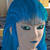Join date: 2 May 2003
Posts: 725
08-29-2005 07:20
Hehe.. Just utilize RPN and not worry about the whole order problem.
Strife Onizuka
Moonchild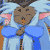Join date: 3 Mar 2004
Posts: 5,887
08-29-2005 11:25
just use xycalc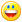oh and it supports RPN too._____________________
Truth is a river that is always splitting up into arms that reunite. Islanded between the arms, the inhabitants argue for a lifetime as to which is the main river.
- Cyril Connolly

Without the political will to find common ground, the continual friction of tactic and counter tactic, only creates suspicion and hatred and vengeance, and perpetuates the cycle of violence.
- James Nachtwey
Keknehv Psaltery
HackerJoin date: 11 Apr 2005
Posts: 1,185
08-30-2005 11:28
xycalc is exceedingly slow... although I can see that RPN would be much easier to code_____________________